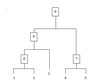hierarchical clustering 

• 上篇博客介绍的层次聚类，尤其是AGNES这一传统的层次聚类算法。这篇博客介绍层次聚类的优化算法。 优化算法 BIRCH算法 BIRCH算法(平衡迭代削减聚类法)：聚类特征使用3元组进行一个簇的相关信息，通过构建满足分枝...
• 主要介绍了Python聚类算法之凝聚层次聚类的原理与具体使用技巧,具有一定参考借鉴价值,需要的朋友可以参考下
• 主要为大家详细介绍了Python实现简单层次聚类算法以及可视化，具有一定的参考价值，感兴趣的小伙伴们可以参考一下
• 7.5 层次聚类方法 2019/3/13 层次聚类 2 层次聚类方法概述 ? 层次聚类方法将数据对象组成一棵聚类 树 ? 根据层次分解是自底向上合并还是 自顶向下分裂进一步分为凝聚的 和分裂的 2019/3/13 层次聚类 3 层次聚类方法...
• 层次聚类算法（HCA）是一种聚类分析方法，它通过层次结构搜索聚类的最佳分布。层次聚类的策略通常有两种类型：使用自下而上的过程进行聚集，而使用自上而下的过程进行分裂。但是，大多数聚类方法都有两个缺点：使用...研究论文
• 层次聚类(AGNES)算法(Python) 是聚类算法的一种，通过计算不同类别数据点间的相似度来创建一棵有层次的嵌套聚类树。在聚类树中，不同类别的原始数据点是树的最低层，树的顶层是一个聚类的根节点。AGNES是常用的一种...
• 自己做的层次聚类 有可视化 有数据集 基于熵特征的聚类
• 这个是一个简单的聚类分析matlab代码实现，通过matlab对数据进行了简单的层次聚类分析matlab
• 层次聚类matlab代码，数据要求字符串格式，数据类型一致，便于计算和使用，提高数据准确度和可用性，简单实用。
• 程序示例，使用C语言，实现不同数据的分类，包括C均值法和层次聚类法。
• %生成20个随机样本 %屏幕输出Q型聚类结果 %屏幕输出R型聚类结果 包含具体聚类步骤和算法，自写函数体
• BIRCH，CURE，ROCK，CHAMELEON。关于层次聚类的papers,5篇英文论文
• 关于层次聚类的一些算法的vvvvvv 懂得而奥迪哥如果该打发额而发阿打发
• 层次聚类的matlab程序，数据来源为80个平面点坐标。
• 凝聚层次聚类算法的改进，张宏，李欣欣，凝聚型层次聚类算法是一个非常有用的聚类算法,它在迭代地凝聚每次接近对直到所有的数据都属于同一个簇。但层次聚类也存在缺点,如�算法理论
• 2.代码支持的凝聚层次聚类算法 通过简要的修改代码中函数的参数，代码可以支持不同的凝聚方法，支持的凝聚方法如下，默认的为代码本身算法： 单连接算法（默认，最近邻聚类算法，最短距离法，最小生成树算法）；全...
• 包括最长、最短欧式距离法、重心法（标准欧式、平方欧式、精度加权）、平均法、权重法等等
• 关于层次聚类(hierarchical clustering)的基本步骤： 1、假设每个样本为一类，计算每个类的距离，也就是相似度 2、把最近的两个合为一新类，这样类别数量就少了一个 3、重新新类与各个旧类(去了那两个合并的类)之间...
• 层次聚类matlab代码层次聚类算法 matlab代码，采用了单链接，完全链接和平均链接算法的层次聚类算法。
• amvidc：基于聚集层次聚类（AHC）的数据聚类算法，该算法使用最小体积增加（MVI）和最小方向变化（MDC）聚类标准
•研究论文
• 基于层次聚类的多维数据分析算法，凝聚层次算法: 初始阶段，将每个样本点分别当做其类簇，然后合并这些原子类簇直至达到预期的类簇数或者其他终止条件。 传统的凝聚层次聚类算法有AGENES，初始时，AGENES将每个样本...
• 基于层次的聚类方法 简介 基于层次的聚类方法 概念 聚合层次聚类 图示 划分层次聚类 图示 基于层次的聚类方法 切割点选取 ...基于层次聚类 ( 聚合层次聚类 ) 算法终止条件 族半径 计算公式 基于层次聚类总结


文章目录
基于层次的聚类方法 简介基于层次的聚类方法 概念聚合层次聚类 图示划分层次聚类 图示基于层次的聚类方法 切割点选取族间距离 概念族间距离 使用到的变量族间距离 最小距离族间距离 最大距离族间距离 中心点距离族间距离 平均距离基于层次聚类 ( 聚合层次聚类 ) 步骤基于层次聚类 ( 聚合层次聚类 ) 算法终止条件族半径 计算公式基于层次聚类总结

基于层次的聚类方法 简介

1 . 基于层次的聚类方法 : 将 数据集样本对象 排列成 聚类树 , 在 指定 的层次 ( 切割点 ) 进行切割 , 切割点 时刻 的聚类分组 , 就是 最终需要的聚类分组 ; 也就是这个切割点的切割的时刻 , 互相关联的样本 , 划分到一个聚类分组中 ;

2 . 基于层次聚类方法 的两种方式 :

① 聚合层次聚类 : 开始时 , 每个对象都是一个聚类分组 ( 原子聚类 ) , 根据 聚类之间的相似性 , 对原子聚类逐渐合并 , 最终会合并成一个聚类 ; 其 本质是 由 多个聚类分组 切割成 成少数 聚类分组 ;

② 划分层次聚类 : 开始时 , 所有的样本都在一个聚类中 , 根据聚类间相似性 , 对聚类进行划分 , 最终 每个样本 都会被划分成一个聚类分组 ( 原子聚类 ) ; 本质是 由 少数 聚类分组 划分成多个 聚类分组 ;

基于层次的聚类方法 概念

1 . 基于层次的聚类方法 概念 : 将数 据集样本对象 排列成 树结构 , 称为 聚类树 , 在指定的层次 ( 步骤 ) 上切割数据集样本 , 切割后时刻的 聚类分组 就是 聚类算法的 聚类结果 ;

2 . 基于层次的聚类方法 : 一棵树可以从叶子节点到根节点 , 也可以从根节点到叶子节点 , 基于这两种顺序 , 衍生出两种方法分支 , 分别是 : 聚合层次聚类 , 划分层次聚类 ;

3 . 聚合层次聚类 ( 叶子节点到根节点 ) : 开始时 , 每个样本对象自己就是一个聚类 , 称为 原子聚类 , 然后根据这些样本之间的 相似性 , 将这些样本对象 ( 原子聚类 ) 进行 合并 ;
常用的聚类算法 : 大多数的基于层次聚类的方法 , 都是 聚合层次聚类 类型的 ; 这些方法从叶子节点到根节点 , 逐步合并的原理相同 ; 区别只是聚类间的相似性计算方式不同 ;

4 . 划分层次聚类 ( 根节点到叶子节点 ) : 开始时 , 整个数据集的样本在一个总的聚类中 , 然后根据样本之间的相似性 , 不停的切割 , 直到完成要求的聚类操作 ;

5 . 算法性能 : 基于层次的聚类方法的时间复杂度为

O

(

N

2

)

O(N^2)

, 如果处理的样本数量较大 , 性能存在瓶颈 ;

聚合层次聚类 图示

1 . 聚合层次聚类 图示 :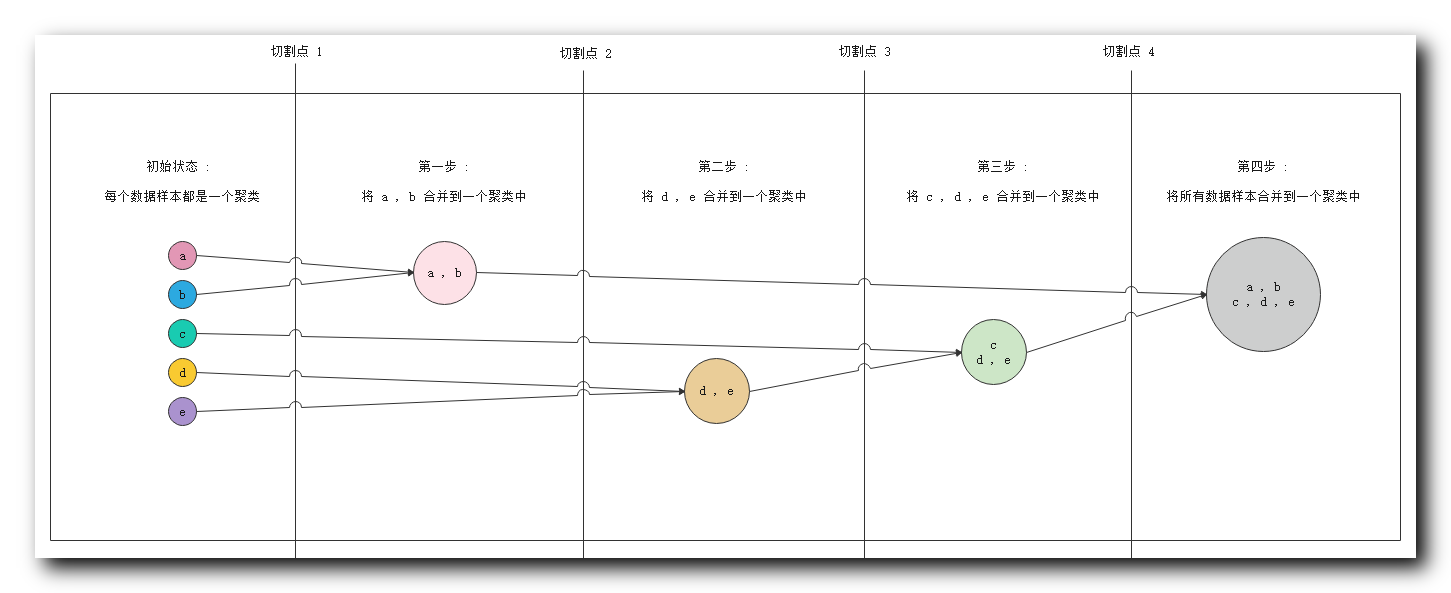① 初始状态 : 最左侧 五个 数据对象 , 每个都是一个聚类 ;
② 第一步 : 分析相似度 , 发现

a

,

b

a , b

相似度很高 , 将

{

a

,

b

}

\{a ,b\}

分到一个聚类中 ;
③ 第二步 : 分析相似度 , 发现

d

,

e

d, e

相似度很高 , 将

{

d

,

e

}

\{d, e\}

分到一个聚类中 ;
④ 第三步 : 分析相似度 , 发现

c

c

与

d

,

e

d,e

相似度很高 , 将

c

c

数据放入

{

d

,

e

}

\{d, e\}

聚类中 , 组成

{

c

,

d

,

e

}

\{c,d, e\}

聚类 ;
⑤ 第四步 : 分析相似度 , 此时要求的相似度很低就可以将不同的样本进行聚类 , 将前几步生成的两个聚类 , 合并成一个聚类

{

a

,

b

,

c

,

d

,

e

}

\{a, b, c, d, e\}

;

2 . 切割点说明 : 实际进行聚类分析时 , 不会将所有的步骤走完 , 这里提供四个切割点 , 聚类算法进行聚类时 , 可以在任何一个切割点停止 , 使用当前的聚类分组当做聚类结果 ;

① 切割点

1

1

: 在切割点

1

1

停止 , 会得到

5

5

个聚类分组 ,

{

a

}

\{a\}

,

{

b

}

\{b\}

,

{

c

}

\{c\}

,

{

d

}

\{d\}

,

{

e

}

\{e\}

;
② 切割点

2

2

: 在切割点

2

2

停止 , 会得到

4

4

个聚类分组 ,

{

a

,

b

}

\{a, b\}

,

{

c

}

\{c\}

,

{

d

}

\{d\}

,

{

e

}

\{e\}

;
③ 切割点

3

3

: 在切割点

3

3

停止 , 会得到

3

3

个聚类分组 ,

{

a

,

b

}

\{a, b\}

,

{

c

}

\{c\}

,

{

d

,

e

}

\{d, e\}

;
④ 切割点

4

4

: 在切割点

4

4

停止 , 会得到

2

2

个聚类分组 ;

{

a

,

b

}

\{a, b\}

,

{

c

,

d

,

e

}

\{c, d, e\}

;
⑤ 走完整个流程 : 会得到

1

1

个聚类分组 ,

{

a

,

b

,

c

,

d

,

e

}

\{a, b ,c, d, e\}

;

划分层次聚类 图示

1 . 划分层次聚类 图示 :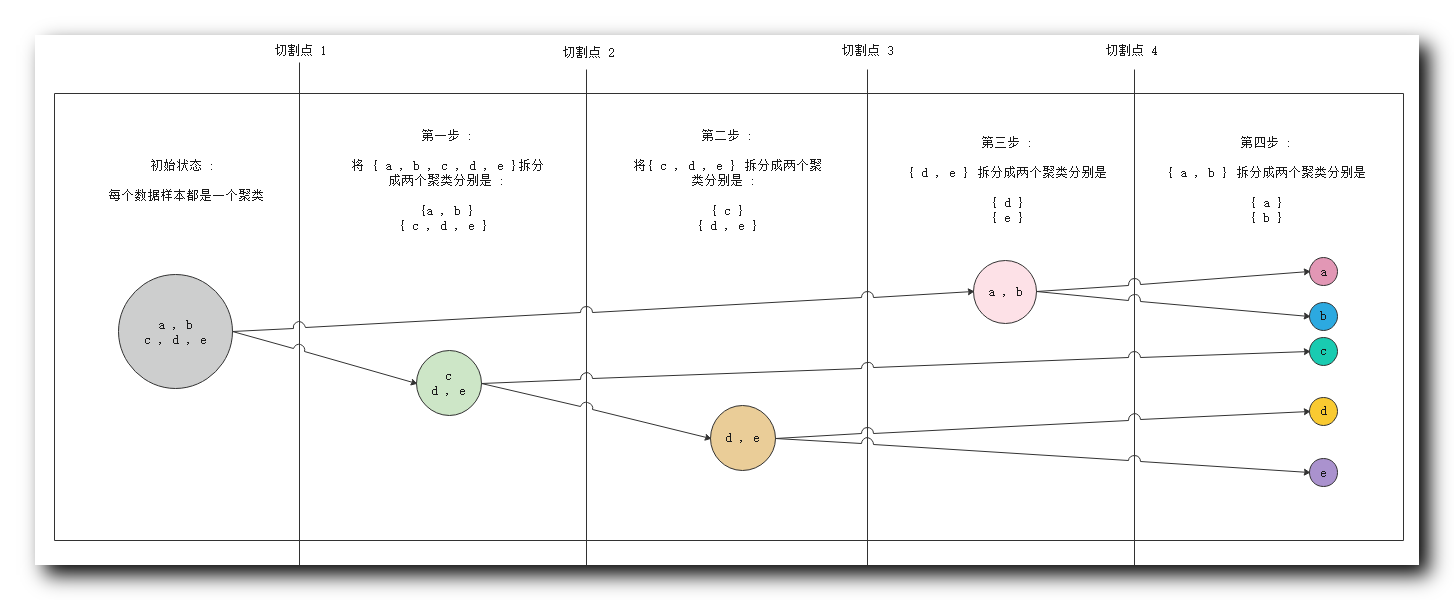① 初始状态 : 最左侧 五个 数据对象 , 属于一个聚类 ;
② 第一步 : 分析相似度 , 切割聚类 , 将

{

c

,

d

,

e

}

\{c,d, e\}

与

{

a

,

b

}

\{a ,b\}

划分成两个聚类 ;
③ 第二步 : 分析相似度 , 将

{

c

,

d

,

e

}

\{c,d, e\}

中的

{

c

}

\{c\}

与

{

d

,

e

}

\{d, e\}

划分成两个聚类 ;
④ 第三步 : 分析相似度 , 将

{

d

,

e

}

\{d, e\}

拆分成

{

d

}

\{d\}

和

{

e

}

\{e\}

两个聚类 ;
⑤ 第四步 : 分析相似度 , 将

{

a

,

b

}

\{a ,b\}

拆分成

{

a

}

\{a\}

和

{

b

}

\{b\}

两个聚类 , 至此所有的数据对象都划分成了单独的聚类 ;

2 . 切割点说明 : 实际进行聚类分析时 , 不会将所有的步骤走完 , 这里提供四个切割点 , 聚类算法进行聚类时 , 可以在任何一个切割点停止 , 使用当前的聚类分组当做聚类结果 ;

① 切割点

1

1

: 在切割点

1

1

停止 , 会得到

1

1

个聚类分组 ,

{

a

,

b

,

c

,

d

,

e

}

\{a, b ,c, d, e\}

;
② 切割点

2

2

: 在切割点

2

2

停止 , 会得到

2

2

个聚类分组 ;

{

a

,

b

}

\{a, b\}

,

{

c

,

d

,

e

}

\{c, d, e\}

;
③ 切割点

3

3

: 在切割点

3

3

停止 , 会得到

3

3

个聚类分组 ,

{

a

,

b

}

\{a, b\}

,

{

c

}

\{c\}

,

{

d

,

e

}

\{d, e\}

\$ ;
④ 切割点

4

4

: 在切割点

4

4

停止 , 会得到

4

4

个聚类分组 ,

{

a

,

b

}

\{a, b\}

,

{

c

}

\{c\}

,

{

d

}

\{d\}

,

{

e

}

\{e\}

;
⑤ 走完整个流程 : 会得到

5

5

个聚类分组 ,

{

a

}

\{a\}

,

{

b

}

\{b\}

,

{

c

}

\{c\}

,

{

d

}

\{d\}

,

{

e

}

\{e\}

;

基于层次的聚类方法 切割点选取

1 . 算法终止条件 ( 切割点 ) : 用户可以指定聚类操作的算法终止条件 , 即上面图示中的切割点 , 如 :

① 聚类的最低个数 : 聚合层次聚类中 ,

n

n

个样本 , 开始有

n

n

个聚类 , 逐步合并 , 聚类个数逐渐减少 , 当聚类个数达到最低值

m

i

n

min

, 停止聚类算法 ;
② 聚类最高个数 : 划分层次聚类中 ,

n

n

个样本 , 开始有

1

1

个聚类 , 逐步划分 , 聚类个数逐渐增加 , 当聚类个数达到最大值

m

a

x

max

, 停止聚类算法 ;
③ 聚类样本的最低半径 : 聚类的数据样本范围不能无限扩大 , 指定一个阈值 , 只有将该阈值内的样本放入一组 ; 半径指的是所有对象距离其平均点的距离 ;

2 . 切割点回退问题 : 切割点一旦确定 , 便无法回退 ; 这里以聚合层次聚类为例 :

① 处于切割点

4

4

: 如已经执行到了步骤三 , 此时处于切割点

4

4

, 聚类分组为

{

a

,

b

}

\{a, b\}

,

{

c

,

d

,

e

}

\{c, d, e\}

;
② 试图回退到 切割点

3

3

: 想要会回退到切割点

3

3

的状态 , 视图将聚类分组恢复成

{

a

,

b

}

\{a, b\}

,

{

c

}

\{c\}

,

{

d

,

e

}

\{d, e\}

;
③ 无法回退 : 该操作是无法实现的 , 聚类分组一旦 合并 或 分裂 , 此时就无法回退 ;

族间距离 概念

族间距离 :

① 作用: 族间距离 , 就是聚类分组之间的距离 , 之前的距离计算都是 样本 之间的距离 , 这里的基于层次聚类时 , 不管是聚合层次聚类 , 还是划分层次聚类 , 其都要进行 聚类分组 间的相似度比较 ,
② 聚合层次聚类 : 是 根据 聚类的族间距离 ( 聚类分组相似性 ) 将不同的聚类分组进行合并 ;
③ 划分层次聚类 : 是 根据 聚类的族间距离 ( 聚类分组相似性 ) 将不同的聚类分组进行划分 ( 拆分 ) ;

族间距离 使用到的变量

公式中 用到的 变量 :

① 样本表示 :

p

p

和

q

q

表示 分别 处于两个聚类分组中的 两个样本 ;
② 样本距离表示 :

d

(

p

,

q

)

d(p, q)

表示

p

p

样本对象 与

q

q

样本对象的距离 ;
③ 聚类 ( 族 ) 表示 :

C

i

C_i

和

C

j

C_j

分别表示两个 聚类 / 族 / 聚类分组 ;
④ 聚类距离表示 :

d

(

C

i

,

C

j

)

d(C_i, C_j)

表示

C

i

C_i

聚类 与

C

j

C_j

聚类 之间的距离 ;
⑤ 聚类中心点 :

m

i

m_i

是

C

i

C_i

聚类的中心点 ,

m

j

m_j

是

C

j

C_j

聚类的中心点 ;
⑥ 样本个数 :

n

i

n_i

是

C

i

C_i

聚类的样本个数 ,

n

j

n_j

是

C

j

C_j

聚类的样本个数 ;

族间距离 最小距离

C

i

,

C

j

C_i \,, C_j

族间距离 最小距离 公式 :

d

m

i

n

(

C

i

,

C

j

)

=

m

i

n

p

∈

C

i

,

q

∈

C

j

d

(

p

,

q

)

d_{min}(C_i , C_j) = min _{p \in C_i , q \in C_j} d(p, q)

d

m

i

n

(

C

i

,

C

j

)

d_{min}(C_i , C_j)

表示两个聚类的最小距离 ;

p

p

是属于

C

i

C_i

聚类中的任意样本 ;

q

q

是属于

C

j

C_j

聚类中的任意样本 ;

总结 : 两个聚类中两个最近的样本之间的距离就是 聚类间的 最小距离 ;

族间距离 最大距离

C

i

,

C

j

C_i \,, C_j

族间距离 最大距离 公式 :

d

m

a

x

(

C

i

,

C

j

)

=

m

a

x

p

∈

C

i

,

q

∈

C

j

d

(

p

,

q

)

d_{max }(C_i , C_j) = max _{p \in C_i , q \in C_j} d(p, q)

d

m

a

x

(

C

i

,

C

j

)

d_{max }(C_i , C_j)

表示两个聚类的最大距离 ;

p

p

是属于

C

i

C_i

聚类中的任意样本 ;

q

q

是属于

C

j

C_j

聚类中的任意样本 ;

总结 : 两个聚类中两个最远的样本之间的距离就是 聚类间的 最大距离 ;

族间距离 中心点距离

C

i

,

C

j

C_i \,, C_j

族间距离 中心点距离 公式 :

d

m

e

a

n

(

C

i

,

C

j

)

=

d

(

m

i

,

m

j

)

d_{mean }(C_i , C_j) = d(m_i, m_j)

d

m

e

a

n

(

C

i

,

C

j

)

d_{mean }(C_i , C_j)

表示两个聚类的 中心点距离 ;

m

i

m_i

是

C

i

C_i

聚类的中心点 ;

m

j

m_j

是

C

j

C_j

聚类的中心点 ;

d

(

m

i

,

m

j

)

d(m_i, m_j)

表示

m

i

m_i

样本 和

m

j

m_j

样本 之间的距离 ;

总结 : 两个聚类中的中心点样本之间的距离就是 聚类间的 中心点距离 ;

族间距离 平均距离

C

i

,

C

j

C_i \,, C_j

族间距离 平均距离 公式 :

d

a

v

g

(

C

i

,

C

j

)

=

1

n

i

n

j

∑

p

∈

C

i

∑

q

∈

C

j

d

(

p

,

q

)

d_{avg}(C_i , C_j) = \frac{1}{n_i n_j}\sum_{p \in C_i}\sum_{q \in C_j} d(p, q)

d

m

e

a

n

(

C

i

,

C

j

)

d_{mean }(C_i , C_j)

表示两个聚类的 中心点距离 ;

p

p

是属于

C

i

C_i

聚类中的任意样本 ;

q

q

是属于

C

j

C_j

聚类中的任意样本 ;

n

i

n_i

是

C

i

C_i

聚类的样本个数 ;

n

j

n_j

是

C

j

C_j

聚类的样本个数 ;

∑

p

∈

C

i

∑

q

∈

C

j

d

(

p

,

q

)

\sum_{p \in C_i}\sum_{q \in C_j} d(p, q)

表示 聚类

C

i

C_i

中每一个点 到 聚类

C

j

C_j

中所有点的距离 , 这里

C

i

C_i

中每个点都对应

n

j

n_j

个距离 ,

n

i

n_i

个点 , 对应

n

i

×

n

j

n_i \times n_j

个距离 ;

总结 : 两个聚类中的 平均距离 就是 聚类间的 所有点的距离的平均距离 ;

基于层次聚类 ( 聚合层次聚类 ) 步骤

聚合层次聚类步骤 :

① 原理 : 根据 聚类分组 的 族间距离 对相似的 聚类分组 进行 逐步合并 ;
② 步骤一 : 每个样本都构成 聚类分组 , 称为 原子聚类 ;
③ 步骤二 : 计算所有 聚类 之间的距离 ; 可以采用 最小距离 , 最大距离 , 中心点距离 , 平均距离 中的一个 ;
④ 步骤三 : 将距离最近的两个 聚类分组 合并 , 聚类的个数 减少

1

1

个 ;
⑤ 步骤四 : 转到 步骤二 计算聚类间距离 , 步骤三 合并近距离聚类 ; 如果满足算法终止条件 , 那么停止聚类 , 否则一直循环迭代 , 最终合并成一个聚类 ;

基于层次聚类 ( 聚合层次聚类 ) 算法终止条件

算法终止条件 : 是由 用户 指定的 , 如 :
① 聚类分组 ( 族 ) 个数 : 当聚类的个数达到阈值 , 算法终止 ;
② 聚类半径 : 每个 聚类的半径 都超过某个阈值 ;

族半径 计算公式

族 ( 聚类 ) 半径计算公式 :

R

=

1

n

∑

i

=

1

n

d

(

p

i

−

m

)

R=\frac{1}{n}\sum _{i=1}^n d(p_i - m)

R

R

表示聚类半径 ;

n

n

表示聚类中的 样本 个数 ;

m

m

代表聚类中心点 ;

d

(

p

i

−

m

)

d(p_i - m)

表示聚类中第

i

i

个样本距离中心点的距离 ;

基于层次聚类总结

1 . 基于层次聚类 的核心 : 是计算 两个 聚类分组 ( 族 ) 之间的距离 , 根据 族间距离 进行 聚类合并 ;

2 . 适用场景 : 如果 每个 聚类 密度差不多 , 族间距离 分离的很清晰 , 那么使用不同的 族间距离 进行聚类 产生的聚类结果 基本一致 ;

3 . 算法缺陷 : 基于层次距离不适用于以下情况 ; 聚类分组 分离的不明显 ; 形状不是球形 , 凹形的 ; 聚类间大小不等 ; 各个聚类间样本密度不同 ;
展开全文聚类 数据挖掘 机器学习
• 针对如何从层次聚类算法得到样本集的多种聚类结果中获得用户最满意的聚类结果,在深入研究聚类有效性的基础上,通过模糊相似性关系刻画聚类的类内致密性和类间分离性,建立了一个新的聚类有效性函数。在人工和实际数据...
• 中科大2019春AI 实验二，包括Kmeans算法，PCA算法和层次聚类算法
• 层次聚类，也称为层次聚类分析，是一种将相似对象分组为聚类的算法。 端点是一组群集，其中每个群集彼此都不相同，并且每个群集内的对象彼此大致相似。 分层聚类技术： 层次聚类是一种流行且易于理解的聚类技术。此...
层次聚类，也称为层次聚类分析，是一种将相似对象分组为聚类的算法。 端点是一组群集，其中每个群集彼此都不相同，并且每个群集内的对象彼此大致相似。
分层聚类技术：
层次聚类是一种流行且易于理解的聚类技术。此聚类技术分为两种类型： 集聚的 分裂性
聚集层次聚类技术：在此技术中，最初，每个数据点都被视为一个单独的聚类。在每次迭代中，相似的群集将与其他群集合并，直到形成一个群集或K个群集。
集聚的基本算法很简单。 计算邻近矩阵
让每个数据点成为一个簇
重复：合并两个最接近的群集并更新邻近矩阵 直到只剩下一个集群 关键操作是计算两个集群的接近度 为了更好地理解，让我们看一下聚集层次聚类技术的图形表示。假设我们有六个数据点{A，B，C，D，E，F}。
步骤1：在第一步中，我们计算单个点的接近度，并将所有六个数据点视为单个簇，如下图所示。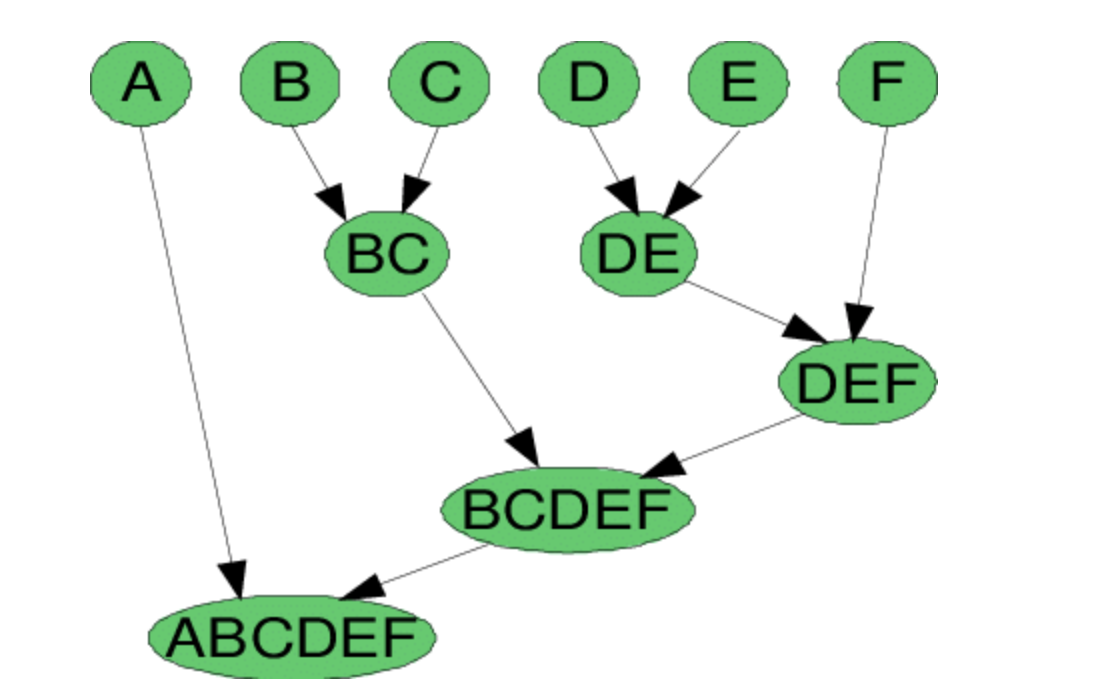步骤2：在第二步中，将相似的群集合并在一起并形成一个群集。 让我们考虑B，C和D，E是在第二步中合并的相似集群。 现在，我们剩下四个群集，分别是A，BC，DE，F。 步骤3：我们再次计算新群集的接近度，并合并相似的群集以形成新群集A，BC，DEF。 步骤4：计算新群集的接近度。 群集DEF和BC相似，合并在一起形成一个新群集。 现在剩下两个簇A，BCDEF。 步骤5：最后，将所有群集合并在一起，形成一个群集。 可以使用树状图来可视化层次聚类技术。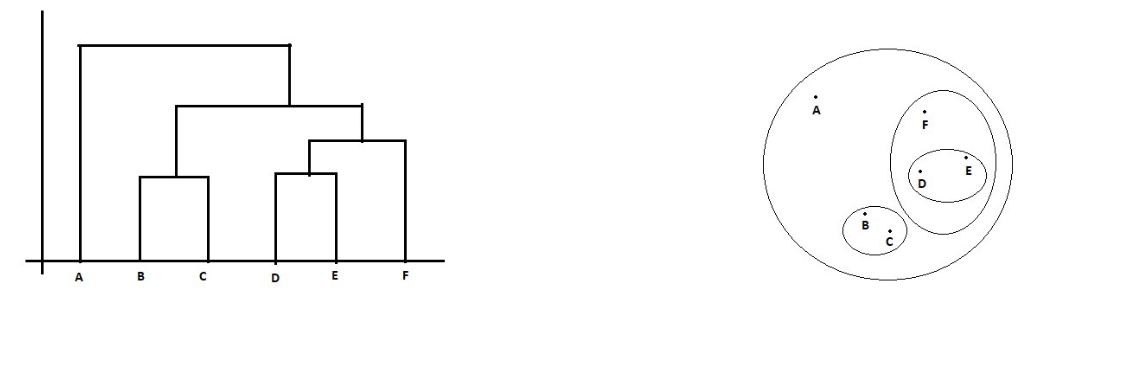2.划分层次聚类技术：由于在现实世界中使用划分层次聚类技术并不多，因此，我将简要介绍划分层次聚类技术。
用简单的话来说，分裂等级聚类与聚集等级聚类恰好相反。在分割层次聚类中，我们将所有数据点视为单个聚类，并且在每次迭代中，我们将数据点与不相似的聚类分开。分离的每个数据点都被视为一个单独的群集。最后，我们将剩下n个群集 。 当我们将单个群集划分为n个群集时，它被称为Divisive Hierarchical群集。
“我们如何计算两个群体之间的相似度？” 计算两个聚类之间的相似性对于合并或划分聚类很重要。有一些方法可用于计算两个聚类之间的相似度：
最小值 最大值 团体平均 Ward 算法
首先是Min也就是最小值算法。
MIN：也可以定义为单链接算法，因为两个群集C1和C2的相似度等于点Pi和Pj之间的相似度的最小值，因此Pi属于C1，Pj属于C2。 从数学上讲，可以写成 Sim（C1，C2）= Min Sim（Pi，Pj），使得Pi∈C1＆Pj∈C2 用简单的话来说，选择两个最接近的点，以使一个点位于聚类1中，另一个点位于聚类2中，并取得它们的相似性并将其声明为两个聚类之间的相似性。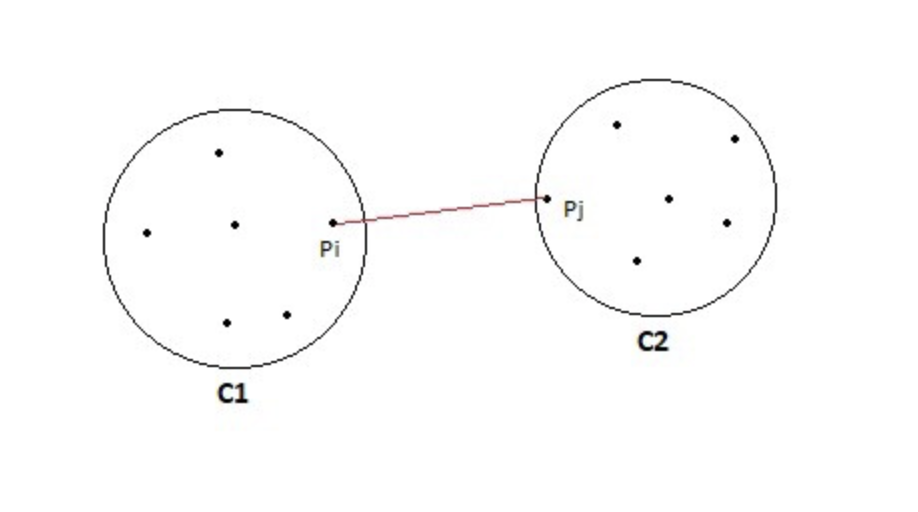MIN的优点： 只要两个簇之间的间隙不小，此方法就可以分离非椭圆形。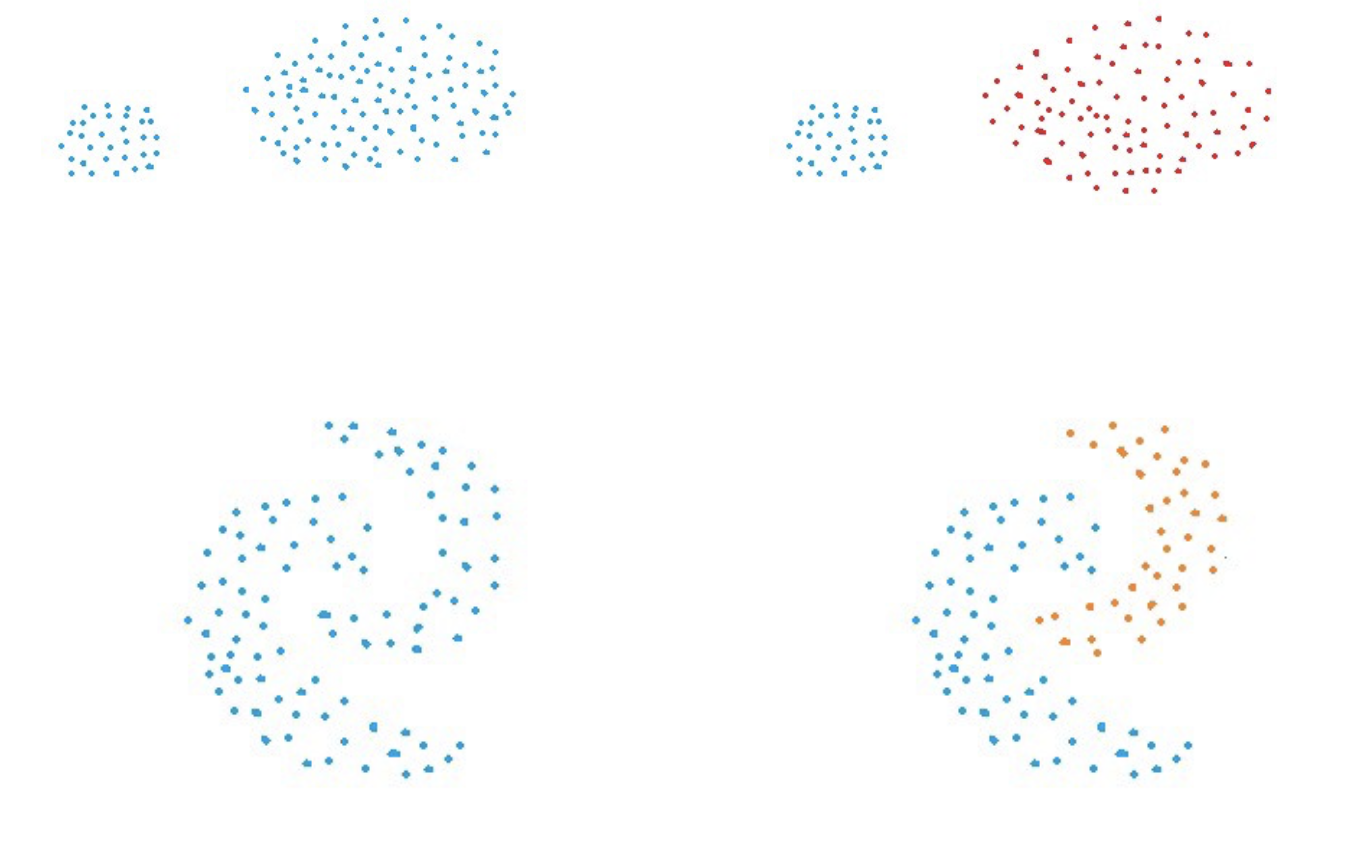MIN的缺点： 如果群集之间存在噪音，则MIN方法无法正确分离群集。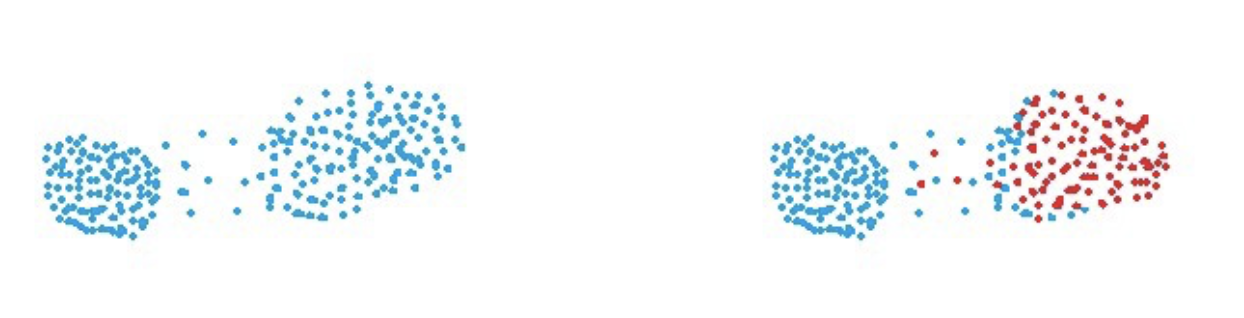下面 是最大值方法： MAX：也称为完全链接算法，与MIN方法完全相反。 两个聚类C1和C2的相似度等于点Pi和Pj之间的相似度的最大值，使得Pi属于C1并且Pj属于C2。 从数学上讲，可以写成 Sim（C1，C2）=最大Sim（Pi，Pj），使得Pi∈C1＆Pj∈C2 用简单的话来说，选择两个最远的点，以便一个点位于群集1中，另一点位于群集2中，并取得它们的相似性并将其声明为两个群集之间的相似性。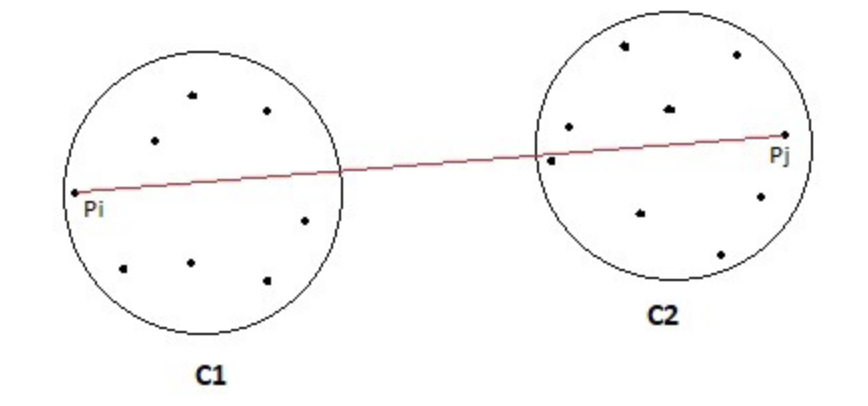MAX的优点： 如果群集之间存在噪声，则MAX方法在隔离群集方面效果很好。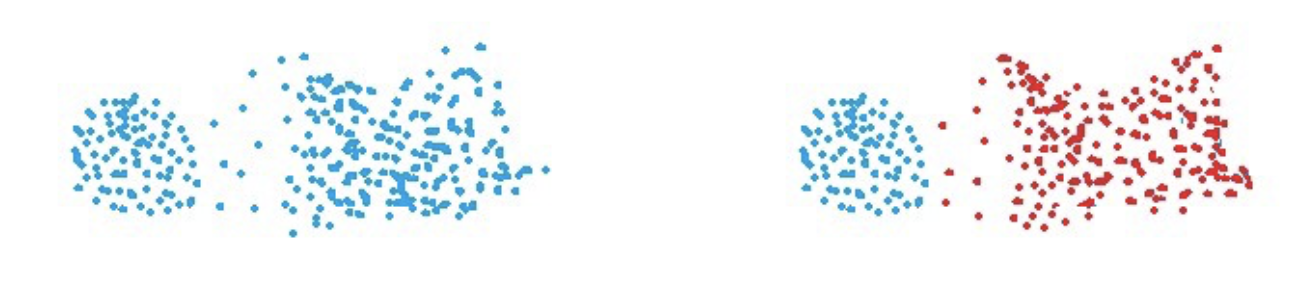最大缺点： 最大方法偏向球状信息团。 最大方法往往会破坏大型群集。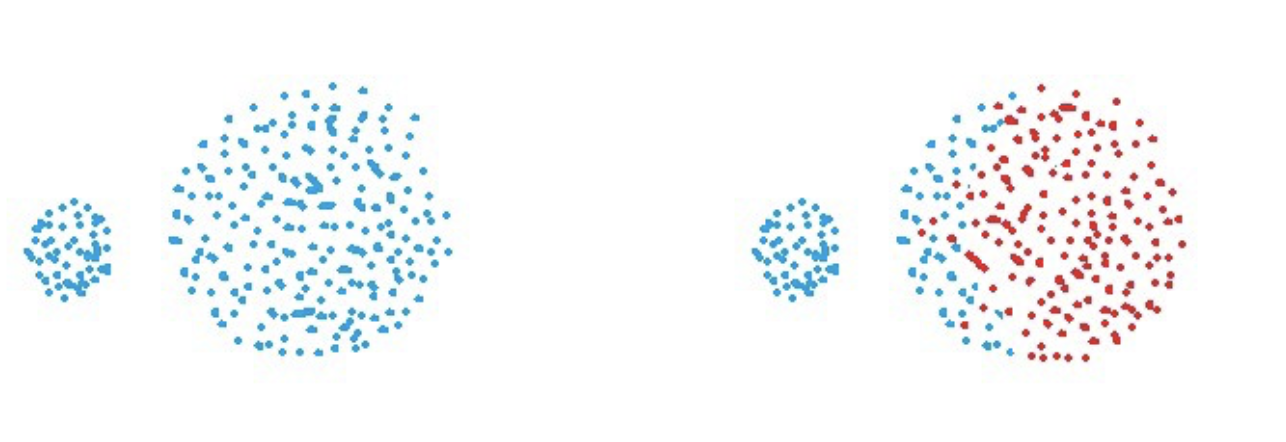Group Average方法 Average Group：取所有成对的点并计算它们的相似度，然后计算相似度的平均值。 从数学上讲，可以写成 sim（C1，C2）= ∑ sim（Pi，Pj）/ | C1 | * | C2 | 其中，Pi∈C1＆Pj∈C2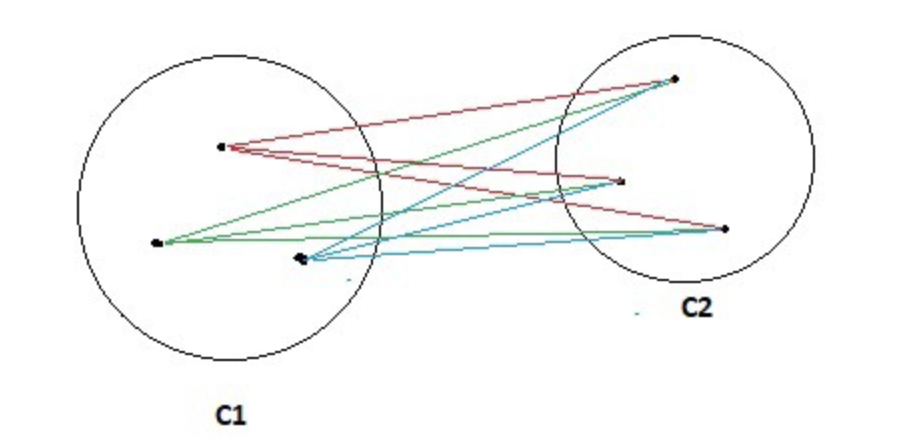团体平均的优点： 如果群集之间存在噪音，则“分组平均值”方法在隔离群集方面效果很好。
团体平均值的缺点： 分组平均法偏向球状信息团。 重心之间的距离：计算两个聚类C1和C2的重心，并将两个重心之间的相似性视为两个聚类之间的相似性。 在现实世界中，这是一种不太流行的技术。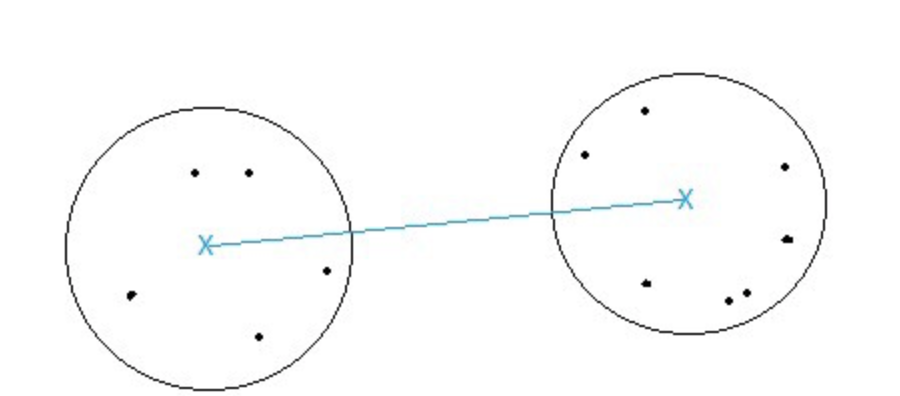沃德的方法：这种计算两个聚类之间相似度的方法与分组平均值完全相同，只是沃德的方法计算的是距离Pi和PJ的平方和。 从数学上讲，可以写成 sim（C1，C2）= ∑（dist（Pi，Pj））²/ | C1 | * | C2 |
沃德方法的优点： 如果群集之间存在噪音，Ward的方法也可以很好地隔离群集。
沃德方法的缺点： 沃德的方法也偏向球状信息团。
展开全文算法...